## Friday, December 26, 2014

### Animations and GIFs using ggplot2

Tracing a regression line Diverging density plots Happy New Year plotHappy New Year everyone! For the last post of the year, I thought I’d have a little fun with the new animation package in R. It’s actually really easy to use. I recently had some fun with it when I presented my research at an electronic poster session, and had an animated movie embedded into the powerpoint.

All of the GIFs above use ggplot and the animation packages. The main idea is to iterate the same plot over and over again, changing incrementally whatever it is that you want to move in the graph, and then save all those plots together into one GIF.

#### 1. Tracing a regression line

Let’s start with first plot that traces a regression line over the scatterplot of the points. We’ll make up some data and fit a loess of two degrees (default). You could easily do this with any kind of regression.

#make up some data
tracedat<-data.frame(x=rnorm(1000,0,1))
tracedat$y<-abs(tracedat$x)*2+rnorm(1000,0,3)

#predict a spline fit and add predicted values to the dataframe
loess_fit <- loess(y ~ x, tracedat)
tracedat$predict_y<-predict(loess_fit) Now let’s make the completed scatterplot and loess line using ggplot. If you need help on how to plot a scatterplot in ggplot, see my post here: ggplot2: Cheatsheet for Scatterplots. It is possible to use stat_smooth() within ggplot to get the loess fit without predicting the values and using geom_line(), but the predicted values are going to make it easier to make the animation. #plot finished scatterplot with loess fit ggplot(tracedat, aes(x,y)) + geom_point() + geom_line(data=tracedat, aes(x,predict_y), color="red", size=1.3) + scale_x_continuous(limits=c(-3, 3)) + scale_y_continuous(limits=c(-10, 10))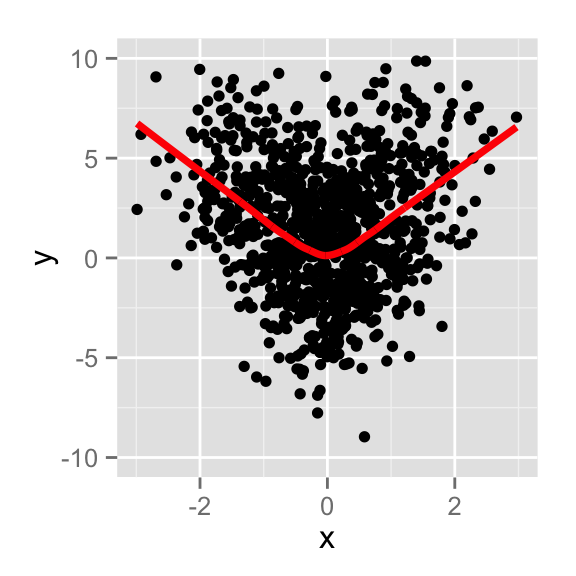Now what we need is for the loess fit to appear bit by bit, so to do this we’ll cut off the dataframe for geom_line for only those x-values up to a certain cutoff x-value (by subsetting the dataframe called tracedat in the geom_line statement). Then we’ll just keep moving that cutoff forward as we iterate over the range of all x-values. First, we will build a function that takes the cutoff value as an argument. Then we can pass whatever value of x we want and it will only graph the line up to that cutoff. Notice how the scatterplot itself, however, is for the full data. For more on how to write functions, see my post about them, here. #function to draw the scatterplot, but the curve fit only up to whatever index we set it at draw.curve<-function(cutoff){ a<-ggplot(tracedat, aes(x,y)) + geom_point() + geom_line(data=tracedat[tracedat$x<cutoff,], aes(x,predict_y), color="red", size=1.3) +
scale_x_continuous(limits=c(-3, 3)) +
scale_y_continuous(limits=c(-10, 10))

print(a)
}

#try it out: draw curve up to cutoff x-value of -2
draw.curve(cutoff=-2)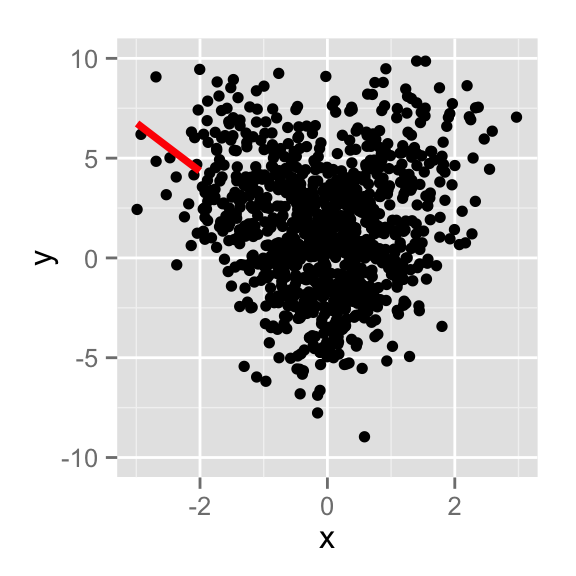Almost done! Now we just need to iterate the draw.curve() function we just created for the full range of the values of x. So we’ll use lapply() to iterate the draw.curve() function over the sequence of i=-3 to 3 (incrementing by .2) and we call the draw.curve() function for each value of i. Finally, we’ll use the saveGIF() function from the animation package to stick all the images together successively into one GIF.

The interval argument tells you how fast the GIF will move from one image to the next, and you can give it a name. If you don’t want the GIF to loop back to the start again, you would add an argument “loop=FALSE” into the function call.

#function to iterate over the full span of x-values
trace.animate <- function() {
lapply(seq(-3,3,.2), function(i) {
draw.curve(i)
})
}

#save all iterations into one GIF
saveGIF(trace.animate(), interval = .2, movie.name="trace.gif")

#### 2. Diverging density plots

The same idea used above is used to make the diverging density plots (plot 2 above). Here we are showing the distribution of scores before some intervention and after the intervention. We need to create the code for a ggplot density plot, and turn it into a function that can take as an argument the variable we want to plot (that way we can use the same code for the “before” intervention plot and the “after” plot. Then to animate, we’ll iterate between them.

We’ll make up some data and plot the “before” plot to start. Notice that we’ll use aes_string rather than simply aes in the ggplot statement in order to be able to pass the data in as an argument when we turn this into a function. More on how to plot distributions using ggplot in my post ggplot2: Cheatsheet for Visualizing Distributions.

It’s important to set the scale for x and y axes so that when we iterate over the two plots, we have the same dimensions each time. The alpha argument in geom_density makes the colors more transparent.

dist.data<-data.frame(base=rnorm(5000, 0, 3),
follow=rnorm(5000, 0, 3),
type=c(rep("Type 1",2500),rep("Type 2",2500)))
dist.data$follow<-ifelse(dist.data$type=="Type 2", dist.data$follow+12, dist.data$follow)

#plot one to make sure it's working. Use aes_string rather than aes
p<-ggplot(dist.data, aes_string("base", fill="type")) +
geom_density(alpha=0.5) +
theme(legend.position="bottom") +
scale_x_continuous(limits=c(-10, 20)) +
scale_y_continuous(limits=c(0, 0.20)) +
scale_fill_manual("", labels=c("Type 1", "Type 2"), values = c("orange","purple")) +
labs(x="Score",y="Density", title="title")

Again, we write two functions: one that draws a density plot based on the arguments passed to it (plot.dens()), and one that iterates over the two different plots (called distdiverge.animate()). In the dist.diverge.animate() function, we pass the plot.item (which is a character class that aes_string will understand as the name of the column in dist.data to plot), and the title, which is also a character class.

#function that plots a density plot with arguments for the variable to plot and the title
plot.dens<-function(plot.item, title.item){
p<-ggplot(dist.data, aes_string(plot.item, fill="type"))+
geom_density(alpha=0.5) +
theme(legend.position="bottom") +
scale_x_continuous(limits=c(-10, 20)) +
scale_y_continuous(limits=c(0, 0.20)) +
scale_fill_manual("", labels=c("Type 1", "Type 2"), values = c("orange","purple"))+
labs(x="Score",y="Density", title=title.item)

print(p)
}

#try it out - plot it for the follow data with the title "After Intervention"
plot.dens(plot.item="follow", title.item="After Intervention")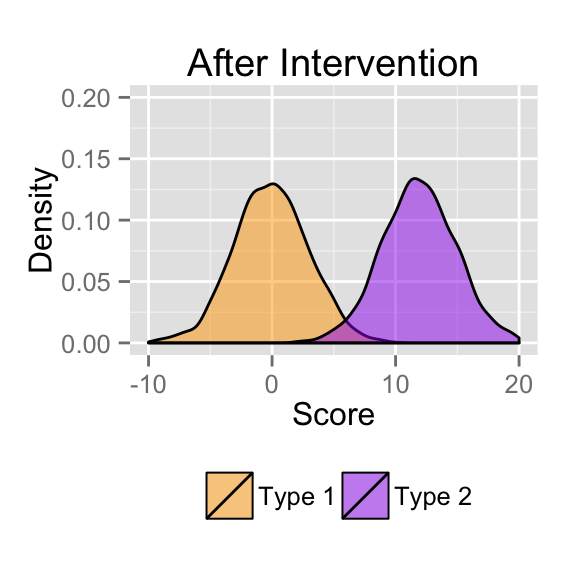#function that iterates over the two different plots
distdiverge.animate <- function() {
items<-c("base", "follow")
titles<-c("Before Intervention","After Intervention")
lapply(seq(1:2), function(i) {
plot.dens(items[i], titles[i])
})
}

We’ll make the interval slower so there’s more time to view each plot before the GIF moves to the next one.

#save in a GIF
saveGIF(distdiverge.animate(), interval = .65, ,movie.name="dist.gif")

#### 3. Happy New Year plot

Finally, to make the fun “Happy 2015” plot, we just make a black background plot, create new random data at every iteration for the snow, and then iterate over the letters of the sign.

Let’s start with the first plot for the letter “H”. We create some data and the objects that will hold the letters of the sign that scrolls through, the colors (I use the colorspace package to pull some colors for me for this), and the x- and y-coordinates for where we want the letters to go. You can think about randomizing the coordinates too. Then we just plot a scatterplot and use annotate to add the letter to it.

#create dataset
happy2015<-data.frame(x=rnorm(500, 0, 1.5), y=rnorm(500, 0, 1.5), z=rnorm(500,0,1.5))

#create objects to hold the letters, colors, and x and y coordinates that we will scroll through
sign<-c("H","A","P","P","Y","2","0","1","5","!!")
colors <- rainbow_hcl(10, c=300)
xcoord<-rep(c(-2, -1, 0, 1, 2),2)
ycoord<-c(2, 1.7, 2.1, 1.5, 2, -.5, 0, -1, -.8, -.7)

We set up the ggplot theme and test the first plot.

#set up the theme in an object (get rid of axes, grids, and legend)
theme.both<- theme(legend.position="none",
panel.background = element_blank(),
axis.ticks = element_blank(),
axis.line = element_blank(),
axis.text.x = element_blank(),
axis.text.y = element_blank(),
plot.background = element_rect(fill = "black"),
panel.grid.major = element_blank(),
panel.grid.minor = element_blank())

#plot the first letter (set index=1 to get the first element of color, letter, and coordinates)
index<-1
ggplot(happy2015, aes(x, y, alpha = z, color=z)) +
geom_point(alpha=0.2) + labs(title="", x="", y="") +
theme.both +
scale_colour_gradient(low = "white", high="lightblue")+
annotate("text", x=xcoord[index], y=ycoord[index], size=15, label=sign[index], color=colors[index])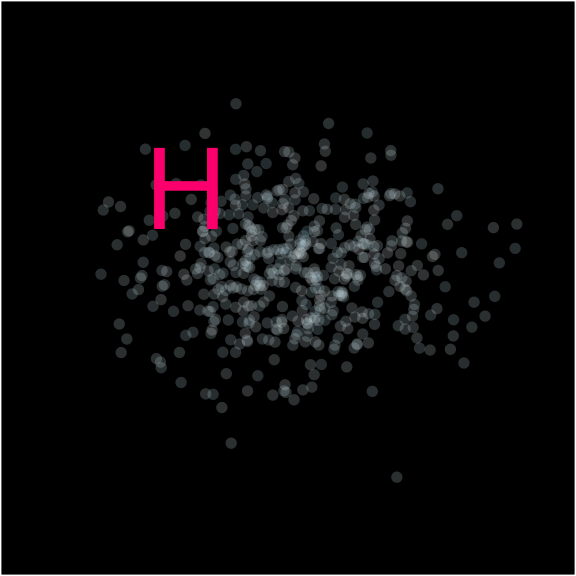Finally, we again go through the structure of two functions - one to draw a plot based on the “index” we give it as an argument, and one to iterate through all the letters using lapply(). Notice we put the dataframe statement in the first function - this will make the scatterplot different every time, rather than stay static, which is more festive (more or less like falling snow). Again, we save it in a GIF, with a slow interval in order to give time to read it.

#set up function to create a new dataset, plot it, and annotate it by an index argument
draw.a.plot<-  function(index){

#make up a new dataframe
happy2015<-data.frame(x=rnorm(500, 0, 1.5), y=rnorm(500, 0, 1.5), z=rnorm(500,0,1.5))

#plot according to the index passed
g<-ggplot(happy2015, aes(x, y, alpha = z, color=z)) +
geom_point(alpha=0.2) + labs(title="", x="", y="") +
theme.both +
scale_colour_gradient(low = "white", high="lightblue")+
annotate("text", x=xcoord[index], y=ycoord[index], size=15, label=sign[index], color=colors[index])

#print out the plot
print(g)
}

#set up function to loop through the draw.a.plot() function
loop.animate <- function() {
lapply(1:length(sign), function(i) {
draw.a.plot(i)
})
}

#save the images into a GIF
saveGIF(loop.animate(), interval = .5, movie.name="happy2015.gif")

Other great sources for learning animations:

Happy New Year everyone! For the last post of the year, I thought I'd have a little fun with the new animation package in R. It's actually really easy to use. I recently had some fun with it when I presented my research at an electronic poster session, and had an animated movie embedded into the powerpoint. All of the GIFs above use ggplot and the animation packages. The main idea is to iterate the same plot over and over again, changing incrementally whatever it is that you want to move in the graph, and then save all those plots together into one GIF. Let's start with first plot that traces a regression line over the scatterplot of the points. We'll make up some data and fit a loess of two degrees (default). You could easily do this with any kind of regression. #make up some data tracedat<-data.frame(x=rnorm(1000,0,1)) tracedat$y<-abs(tracedat$x)*2+rnorm(1000,0,3) #predict a spline fit and add predicted values to the dataframe loess_fit <- loess(y ~ x, tracedat) tracedat$predict_y<-predict(loess_fit) Now let's make the completed scatterplot and loess line using ggplot. If you need help on how to plot a scatterplot in ggplot, see my post here: [ggplot2: Cheatsheet for Scatterplots](http://rforpublichealth.blogspot.com/2013/11/ggplot2-cheatsheet-for-scatterplots.html). It is possible to use **stat_smooth()** within **ggplot** to get the loess fit without predicting the values and using **geom_line()**, but the predicted values are going to make it easier to make the animation. #plot finished scatterplot with loess fit ggplot(tracedat, aes(x,y)) + geom_point() + geom_line(data=tracedat, aes(x,predict_y), color="red", size=1.3) + scale_x_continuous(limits=c(-3, 3)) + scale_y_continuous(limits=c(-10, 10)) Now what we need is for the loess fit to appear bit by bit, so to do this we'll cut off the dataframe for **geom_line** for only those x-values up to a certain cutoff x-value (by subsetting the dataframe called tracedat in the **geom_line** statement). Then we'll just keep moving that cutoff forward as we iterate over the range of all x-values. First, we will build a function that takes the cutoff value as an argument. Then we can pass whatever value of x we want and it will only graph the line up to that cutoff. Notice how the scatterplot itself, however, is for the full data. For more on how to write functions, see my post about them, [here](http://rforpublichealth.blogspot.com/2014/06/how-to-write-and-debug-r-function.html). #function to draw the scatterplot, but the curve fit only up to whatever index we set it at #try it out: draw curve up to cutoff x-value of -2 draw.curve(cutoff=-2) Almost done! Now we just need to iterate the **draw.curve()** function we just created for the full range of the values of x. So we'll use **lapply()** to iterate the **draw.curve()** function over the sequence of i=-3 to 3 (incrementing by .2) and we call the **draw.curve()** function for each value of i. Finally, we'll use the saveGIF() function from the animation package to stick all the images together successively into one GIF. The interval argument tells you how fast the GIF will move from one image to the next, and you can give it a name. If you don't want the GIF to loop back to the start again, you would add an argument "loop=FALSE" into the function call. #function to iterate over the full span of x-values trace.animate <- function() { lapply(seq(-3,3,.2), function(i) { draw.curve(i) }) } #save all iterations into one GIF saveGIF(trace.animate(), interval = .2, movie.name="trace.gif") ####2. Diverging density plots The same idea used above is used to make the diverging density plots (plot 2 above). Here we are showing the distribution of scores before some intervention and after the intervention. We need to create the code for a ggplot density plot, and turn it into a function that can take as an argument the variable we want to plot (that way we can use the same code for the "before" intervention plot and the "after" plot. Then to animate, we'll iterate between them. We'll make up some data and plot the "before" plot to start. Notice that we'll use **aes_string** rather than simply **aes** in the ggplot statement in order to be able to pass the data in as an argument when we turn this into a function. More on how to plot distributions using **ggplot** in my post [ggplot2: Cheatsheet for Visualizing Distributions](http://rforpublichealth.blogspot.ie/2014/02/ggplot2-cheatsheet-for-visualizing.html). It's important to set the scale for x and y axes so that when we iterate over the two plots, we have the same dimensions each time. The alpha argument in **geom_density** makes the colors more transparent. dist.data<-data.frame(base=rnorm(5000, 0, 3), follow=rnorm(5000, 0, 3), type=c(rep("Type 1",2500),rep("Type 2",2500))) dist.data$follow<-ifelse(dist.data$type=="Type 2", dist.data$follow+12, dist.data\$follow) #plot one to make sure it's working. Use aes_string rather than aes p<-ggplot(dist.data, aes_string("base", fill="type")) + geom_density(alpha=0.5) + theme(legend.position="bottom") + scale_x_continuous(limits=c(-10, 20)) + scale_y_continuous(limits=c(0, 0.20)) + scale_fill_manual("", labels=c("Type 1", "Type 2"), values = c("orange","purple")) + labs(x="Score",y="Density", title="title") Again, we write two functions: one that draws a density plot based on the arguments passed to it (**plot.dens()**), and one that iterates over the two different plots (called **distdiverge.animate()**). In the **dist.diverge.animate()** function, we pass the plot.item (which is a character class that aes_string will understand as the name of the column in dist.data to plot), and the title, which is also a character class. #function that plots a density plot with arguments for the variable to plot and the title plot.dens<-function(plot.item, title.item){ p<-ggplot(dist.data, aes_string(plot.item, fill="type"))+ geom_density(alpha=0.5) + theme(legend.position="bottom") + scale_x_continuous(limits=c(-10, 20)) + scale_y_continuous(limits=c(0, 0.20)) + scale_fill_manual("", labels=c("Type 1", "Type 2"), values = c("orange","purple"))+ labs(x="Score",y="Density", title=title.item) print(p)} #try it out - plot it for the follow data with the title "After Intervention" plot.dens(plot.item="follow", title.item="After Intervention") #function that iterates over the two different plots distdiverge.animate <- function() { items<-c("base", "follow") titles<-c("Before Intervention","After Intervention") lapply(seq(1:2), function(i) { plot.dens(items[i], titles[i]) })} We'll make the interval slower so there's more time to view each plot before the GIF moves to the next one. #save in a GIF saveGIF(distdiverge.animate(), interval = .65, ,movie.name="dist.gif") ####3. Happy New Year plot Finally, to make the fun "Happy 2015" plot, we just make a black background plot, create new random data at every iteration for the snow, and then iterate over the letters of the sign. Let's start with the first plot for the letter "H". We create some data and the objects that will hold the letters of the sign that scrolls through, the colors (I use the colorspace package to pull some colors for me for this), and the x- and y-coordinates for where we want the letters to go. You can think about randomizing the coordinates too. Then we just plot a scatterplot and use annotate to add the letter to it. #create dataset happy2015<-data.frame(x=rnorm(500, 0, 1.5), y=rnorm(500, 0, 1.5), z=rnorm(500,0,1.5)) #create objects to hold the letters, colors, and x and y coordinates that we will scroll through sign<-c("H","A","P","P","Y","2","0","1","5","!!") colors <- rainbow_hcl(10, c=300) xcoord<-rep(c(-2, -1, 0, 1, 2),2) ycoord<-c(2, 1.7, 2.1, 1.5, 2, -.5, 0, -1, -.8, -.7) We set up the ggplot theme and test the first plot. #set up the theme in an object (get rid of axes, grids, and legend) theme.both<- theme(legend.position="none", panel.background = element_blank(), axis.ticks = element_blank(), axis.line = element_blank(), axis.text.x = element_blank(), axis.text.y = element_blank(), plot.background = element_rect(fill = "black"), panel.grid.major = element_blank(), panel.grid.minor = element_blank()) #plot the first letter (set index=1 to get the first element of color, letter, and coordinates) index<-1 ggplot(happy2015, aes(x, y, alpha = z, color=z)) + geom_point(alpha=0.2) + labs(title="", x="", y="") + theme.both + scale_colour_gradient(low = "white", high="lightblue")+ annotate("text", x=xcoord[index], y=ycoord[index], size=15, label=sign[index], color=colors[index]) Finally, we again go through the structure of two functions - one to draw a plot based on the "index" we give it as an argument, and one to iterate through all the letters using lapply(). Notice we put the dataframe statement in the first function - this will make the scatterplot different every time, rather than stay static, which is more festive (more or less like falling snow). Again, we save it in a GIF, with a slow interval in order to give time to read it. #set up function to create a new dataset, plot it, and annotate it by an index argument draw.a.plot<- function(index){ #make up a new dataframe happy2015<-data.frame(x=rnorm(500, 0, 1.5), y=rnorm(500, 0, 1.5), z=rnorm(500,0,1.5)) #plot according to the index passed g<-ggplot(happy2015, aes(x, y, alpha = z, color=z)) + geom_point(alpha=0.2) + labs(title="", x="", y="") + theme.both + scale_colour_gradient(low = "white", high="lightblue")+ annotate("text", x=xcoord[index], y=ycoord[index], size=15, label=sign[index], color=colors[index]) #print out the plot print(g)} #set up function to loop through the draw.a.plot() function loop.animate <- function() { lapply(1:length(sign), function(i) { draw.a.plot(i) })} #save the images into a GIF saveGIF(loop.animate(), interval = .5, movie.name="happy2015.gif")

#### 8 comments:

1.Nice examples! And happy new year to you too. But I find that having to specify a function in saveGIF() is a bind. Not to mention GIF limitations. So I wrote up some nuts-n-bolts stop-frame animation examples in R and Stata at my website http://www.animatedgraphs.co.uk I thought animation would prove popular but interactivity is where it's at. Still, y'all might find some useful ideas there.

1.Thanks Robert. Your site is great! Nice job on the recreated gapminder.

2.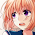An usefull post,thx to admin for the article

3.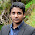This post is very useful and helpful. I like your posts. I always follow your Posts. I hope, in the future, you will present us with such important posts.
Computer tech BD IT Tips Youtube Tutorial

4.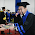Thank you so much its helpful, i want to tell my friend, she will happy to read this article

visit our home

Internet Gratis buat Download Anime | Haruhi Blog
Baca Berita Anime Manga Jepang

5.Nice, Thank to admin for the article

6.Then what is the advantage (or dissadvantage) of using the package gganimate?

7.Awesome post. I'm trying to adapt the code from the first example (Tracing a regression line) to trace several trend lines in a single plot. Instead of geom_point(), I am using geom_blank() because I don't want the points to show. I am using multiple geom_line() statements to include several lines on the plot. However, I am having trouble showing a legend and changing the xlab. Any hints?

Note: Only a member of this blog may post a comment.